Courses

# Review of Spectral Characteristics of Periodic and Non Periodic Signals Electronics and Communication Engineering (ECE) Notes | EduRev

## Communication Theory

Created by: Machine Experts

## Electronics and Communication Engineering (ECE) : Review of Spectral Characteristics of Periodic and Non Periodic Signals Electronics and Communication Engineering (ECE) Notes | EduRev

The document Review of Spectral Characteristics of Periodic and Non Periodic Signals Electronics and Communication Engineering (ECE) Notes | EduRev is a part of the Electronics and Communication Engineering (ECE) Course Communication Theory.
All you need of Electronics and Communication Engineering (ECE) at this link: Electronics and Communication Engineering (ECE)

Introduction:

In electronics, a signal is an electric current or electromagnetic field used to convey data from one place to another. The simplest form of signal is a direct current (DC) that is switched on and off; this is the principle by which the early telegraph worked. More complex signals consist of an alternating-current (AC) or electromagnetic carrier that contains one or more data streams.

Modulation:

Modulation is the addition of information (or the signal) to an electronic or optical signal carrier. Modulation can be applied to direct current (mainly by turning it on and off), to alternating current, and to optical signals. One can think of blanket waving as a form of modulation used in smoke signal transmission (the carrier being a steady stream of smoke). Morse code, invented for telegraphy and still used in amateur radio, uses a binary (two-state)digital code similar to the code used by modern computers. For most of radio and telecommunication today, the carrier is alternating current (AC) in a given range of frequencies. Common modulation methods include:

• Amplitude modulation (AM), in which the voltage applied to the carrier is varied over time
• Frequency modulation (FM), in which the frequency of the carrier waveform is varied in small but meaningful amounts
• Phase modulation (PM), in which the natural flow of the alternating current waveform is delayed temporarily

Classification of Signals:

Some important classifications of signals

• Analog vs. Digital signals: as stated in the previous lecture, a signal with a magnitude that may take any real value in a specific range is called an analog signal while a signal with amplitude that takes only a finite number of values is called a digital signal.
• Continuous-time vs. discrete-time signals: continuous-time signals may be analog or digital signals such that their magnitudes are defined for all values of t, while discrete-time signal are analog or digital signals with magnitudes that are defined at specific instants of time only and are undefined for other time instants.
• Periodic vs. aperiodic signals: periodic signals are those that are constructed from a specific shape that repeats regularly after a specific amount of time T0, [i.e., a periodic signal f(t) with period T0 satisfies f(t) = f(t+nT0) for all integer values of n], while aperiodic signals do not repeat regularly.
• Deterministic vs. probabilistic signals: deterministic signals are those that can be computed beforehand at any instant of time while a probabilistic signal is one that is random and cannot be determined beforehand.
• Energy vs. Power signals: as described below.

Energy and Power Signals

The total energy contained in and average power provided by a signal f(t) (which is a function of time) are defined as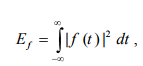and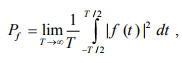respectively.

For periodic signals, the power P can be computed using a simpler form based on the periodicity of the signal as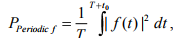where T here is the period of the signal and t0 is an arbitrary time instant that is chosen to simply the computation of the integration (to reduce the functions you have to integrate over one period).

Classification of Signals into Power and Energy Signals

Most signals can be classified into Energy signals or Power signals. A signal is classified into an energy or a power signal according to the following criteria

a) Energy Signals: an energy signal is a signal with finite energy and zero average power (0 ≤ E <∞ , P = 0),

b) Power Signals: a power signal is a signal with infinite energy but finite average power (0 < P < ∞, E→∞ ).

1. The square root of the average power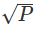of a power signal is what is usually defined as the RMS value of that signal.
2. Your book says that if a signal approaches zero as t approaches then the signal is an energy signal. This is in most cases true but not always as you can verify in part (d) in the following example.
3. All periodic signals are power signals (but not all non–periodic signals are energy signals).
4. Any signal f that has limited amplitude (| f | < ∞ ) and is time limited (f = 0 for | t | > t0 for some t0 > 0) is an energy signal as in part (g) in the following example.

Exercise 1: determine if the following signals are Energy signals, Power signals, or neither, and evaluate E and P for each signal.

a)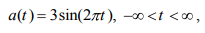This is a periodic signal, so it must be a power signal. Let us prove it.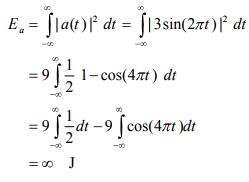Notice that the evaluation of the last line in the above equation is infinite because of the first term. The second term has a value between –2 to 2 so it has no effect in the overall value of the energy.

Since a(t) is periodic with period T = 2 π/2π = 1 second, we get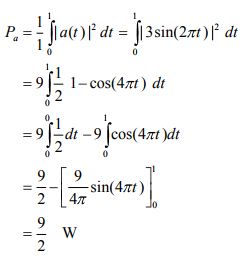So, the energy of that signal is infinite and its average power is finite (9/2). This means that it is a power signal as expected. Notice that the average power of this signal is as expected (square of the amplitude divided by 2)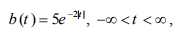Let us first find the total energy of the signal.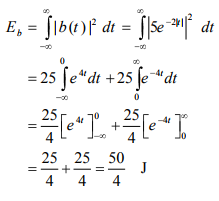The average power of the signal is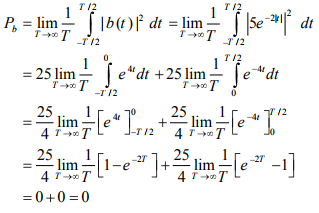So, the signal b(t) is definitely an energy signal. So, the energy of that signal is infinite and its average power is finite (9/2). This means that it is a power signal as expected. Notice that the average power of this signal is as expected (the square of the amplitude divided by 2)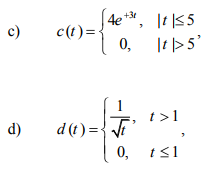Let us first find the total energy of the signal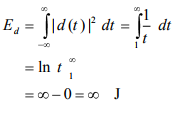So, this signal is NOT an energy signal. However, it is also NOT a power signal since its average power as shown below is zero.

The average power of the signal is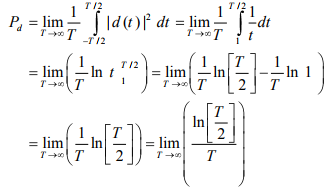Using Le‘hopital‘s rule, we see that the power of the signal is zero. That is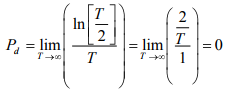So, not all signals that approach zero as time approaches positive and negative infinite is an energy signal. They may not be power signals either.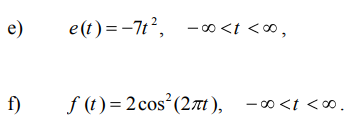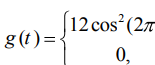t),  - 8 < t < 31 elsewhere

Offer running on EduRev: Apply code STAYHOME200 to get INR 200 off on our premium plan EduRev Infinity!

,

,

,

,

,

,

,

,

,

,

,

,

,

,

,

,

,

,

,

,

,

;